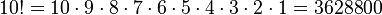# Symmetric group:S10

This is defined as the symmetric group on a set of size$10$, which for concreteness we can take as the set$\{ 1,2,3,4,5,6,7,8,9,10 \}$. In other words, it is the group of all permutations on nine elements under composition.
order (number of elements, equivalently, cardinality or size of underlying set) 3628800 groups with same order$10! = 10 \cdot 9 \cdot 8 \cdot 7 \cdot 6 \cdot 5 \cdot 4 \cdot 3 \cdot 2 \cdot 1 = 3628800$